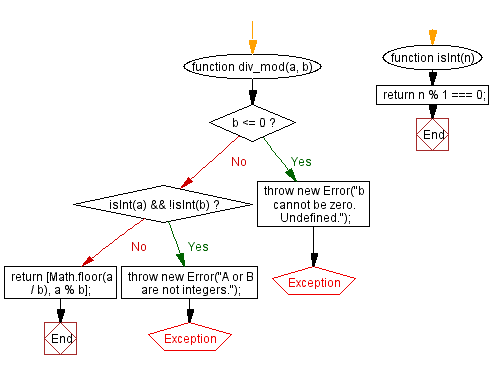# JavaScript: Calculate the divisor and modulus of two integers

## JavaScript Math: Exercise-46 with Solution

Write a JavaScript function to calculate the divisor and modulus of two integers.

Sample Solution:-

HTML Code:

``````<!DOCTYPE html>
<html>
<meta charset="utf-8">
<title>JavaScript function to calculate the divisor and modulus of two integers</title>
<body>

</body>
</html>
```
```

JavaScript Code:

``````function div_mod(a, b)
{
if (b <= 0)
throw new Error("b cannot be zero. Undefined.");
if (isInt(a) && !isInt(b))
throw new Error("A or B are not integers.");
return [Math.floor(a / b), a % b];
}
function isInt(n) {
return n % 1 === 0;
}
console.log(div_mod(17, 4));
```
```

Sample Output:

```[4,1]
```

Flowchart:Live Demo:

See the Pen javascript-math-exercise-46 by w3resource (@w3resource) on CodePen.

Improve this sample solution and post your code through Disqus

What is the difficulty level of this exercise?

Test your Programming skills with w3resource's quiz.

﻿